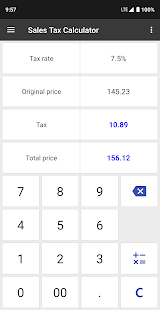ClevCalc Calculator

This calculator allows you to easily handle all the calculations necessary for everyday life with a single application. A calculator app with a clean interface and practical functions!

List of calculators currently supported:

1. General Calculator
• Supports the four fundamental arithmetic operations, square, formula’s parenthesis, and simple engineering functions.
• Quick and easy.
• Possible to modify the formula during the input.
• History available.

2. Unit Converter
• Supports length, weight, width, volume, time, temperature, pressure, speed, fuel efficiency, and the amount of data.
• Supports all unit conversions commonly used in everyday life.

3. Currency Converter
• Supports 106 currencies in the world, including dollar, euro, yen, yuan, etc.
• Automatically calculates using the real time exchange rate.

4. Discount Calculator
• Get a discount price by entering the original price and the discount rate.

5. World Time Converter
• Transforms the time of 400 or more cities around the world.
• Daylight savings time will also be reflected in this calculation.

6. GPA Calculator
• You can correctly calculate your GPA!

7. Ovulation Calculator
• Calculates the time of ovulation and fertility using the menstrual cycle!
• You can also create notes by date.

8. Date Calculator
• A feature that calculates the specific date or anniversary to be remembered!

9. Unit Price Calculator
• Enter the price and the quantity and you will get the unit price.
• You can compare unit prices of various goods.

10. Health Calculator
• You can measure the body mass index (BMI) and the basal metabolic rate (BMR).

11. Fuel Efficiency Calculator
• Enter the amount of fuel used to get the fuel efficiency.

12. Automobile Fuel Cost Calculator
• You can calculate the fuel costs required for driving a car or traveling.
• Enter a distance and a fuel efficiency to get a fuel cost.

• Converts between decimal and hexadecimal with ease and convenience.

14. Sales Tax Calculator
• Get a total price by entering the original price and the tax rate.

15. Loan Calculator
• You can calculate the total interest and total payments by entering the loan principal and interest rate.

Screenshots

•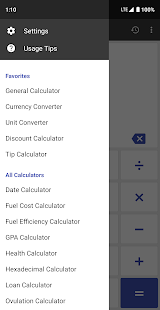•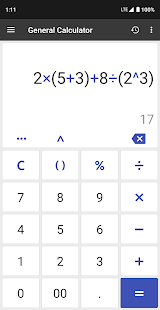•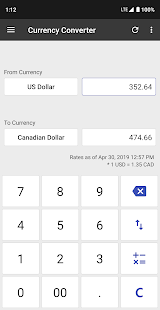•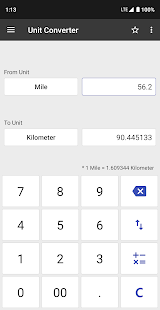•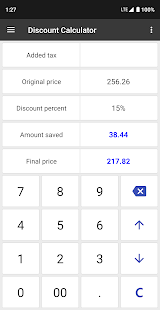•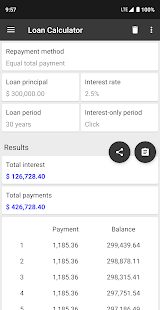•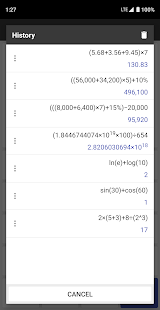•